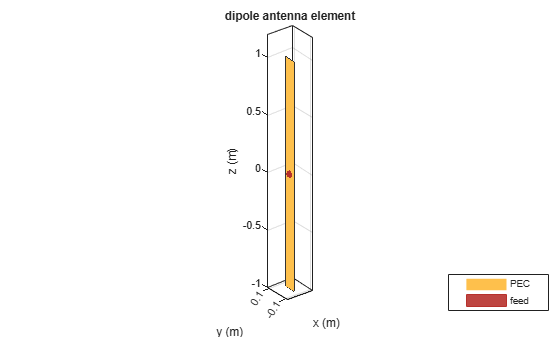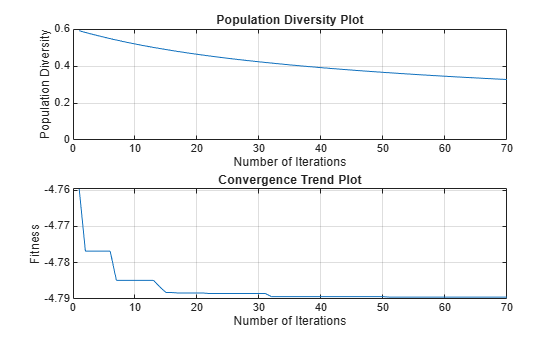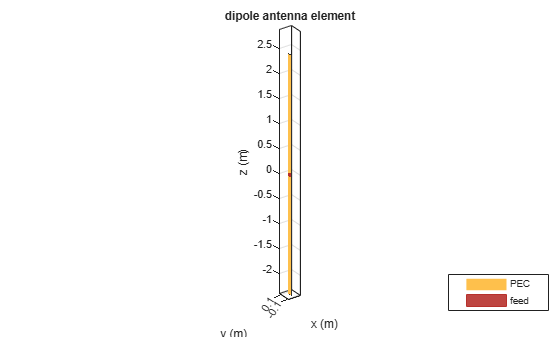optimize

Optimize antenna or array using SADEA optimizer

Description

optimizedelement = optimize(element,frequency,objectivefunction,propertynames,bounds) optimizes the antenna or the array at the specified frequency using the specified objective function and the antenna or array properties and their bounds.

example

optimizedelement = optimize(___,Name,Value) optimizes the antenna or the array using additional name value pairs.

Examples

collapse all

Create and view a default dipole antenna.

ant = dipole;
show(ant)Maximize the gain of the antenna by changing the antenna length from 3 m to 7 m and the width from 0.11 m to 0.13 m.

Optimize the antenna at a frequency of 75 MHz.

optAnt = optimize(ant, 75e6, 'maximizeGain', ...
{'Length', 'Width'}, {3 0.11; 7 0.13})optAnt =
dipole with properties:

Length: 4.7585
Width: 0.1129
FeedOffset: 0
Conductor: [1x1 metal]
Tilt: 0
TiltAxis: [1 0 0]

show(optAnt)Input Arguments

collapse all

Antenna or array element, specified as an antenna object from the Antenna Catalog or array object from the Array Catalog.

Frequency of the antenna or array analysis during optimization, specified as a nonnegative scalar in hertz.

Data Types: double

Objective of antenna or array optimization, specified as one of the following:

• 'maximizeGain' — Maximize the gain of the given antenna or array element

• 'fronttoBackRatio' — Increase the front-lobe-to-back-lobe ratio of the antenna or array element

• 'maximizeBandwidth' — Maximize the operation bandwidth of the antenna or array element. Use this objective function for optimizing antennas or arrays for wideband applications.

• 'minimizeBandwidth' — Minimize the operation bandwidth of the antenna or array element. Use this objective function for optimizing antennas or arrays for narrowband applications.

• 'maximizeSLL' — Maximize the ratio between the front lobe and the first side lobes of the antenna or array pattern.

• 'minimizeArea' — Minimizes the maximum area occupied by the antenna or the array element. If the dimension of the element in the array is smaller than the aperture, the objective function minimizes the array aperture.

Data Types: string | char

Properties of optimizing antenna or array, specified as a cell array of character vectors. The property names are selected as the design variables in optimization.

Data Types: cell

Lower and upper bounds of design variables, specified as a two-row cell array.

Data Types: double

Name-Value Arguments

Specify optional comma-separated pairs of Name,Value arguments. Name is the argument name and Value is the corresponding value. Name must appear inside quotes. You can specify several name and value pair arguments in any order as Name1,Value1,...,NameN,ValueN.

Example: optAnt = optimize(ant, 75e6, 'maximizeGain',{'Length', 'Width'}, {3 0.11; 7 0.13})

Antenna or array optimization constraints, specified as the comma-separated pair consisting of 'Constraints' and a cell array of strings or character vectors. Each character vector or string must be of the form: (analysis function) (inequality sign) (value). You can specify any of the following analysis functions:

• 'Area' in meter square

• 'Volume' in meter cube

• 'S11' in dB

• 'Gain' in dBi

• 'F/B' in dBi

• 'SLL' in dBi

The inequality signs '<' or '>' and the values specifies the analysis function limits. For example, Area < 0.03 indicates that the area of the optimizing antenna must be lesser than 0.03 square meter.

Example: 'Constraints',{Area<0.03}

Data Types: char | string

Weight or penalty of each constraint function, specified as the comma-separated pair consisting of 'Weights' and a vector of positive integers in the range (1,100). If the penalty is set to high, a higher priority is given to the constraint function in case of multiple constraint optimization. All constraint functions are weighted equally by default.

Example: 'Weights',8

Data Types: double

Range of frequencies for vector frequency analysis like S-parameters, specified as the comma-separated pair consisting of 'FrequencyRange' and a vector of nonnegative numbers with each element unit in hertz.

The default frequency range is obtained from the center frequency considering a bandwidth of less than 10 percent.

Example: 'FrequencyRange',linspace(1e9,2e9,10)

Data Types: double

Reference impedance of antenna or array being optimized, specified as the comma-separated pair consisting of 'ReferenceImpedance' and a scalar in ohms

Example: 'ReferenceImpedance',50

Data Types: double

Azimuth and elevation of main lobe of antenna or array being optimized, specified as the comma-separated pair consisting of 'MainLobeDirection' and a two-element vector with each element unit in degrees. The first element represents azimuth and the second element represents elevation.

Example: 'MainLobeDirection',[20 30]

Data Types: double

Number of iterations to run the optimizer after you build the model, specified as the comma-separated pair consisting of 'Iterations' and a positive scalar.

Example: 'Iterations',40

Data Types: double

Use Parallel Computing Toolbox during optimization, specified as the comma-separated pair consisting of 'UseParallel' and true or false.

Example: 'UseParallel',true

Data Types: logical

Enable mutual coupling of elements in an array during optimization, specified as the comma-separated pair consisting of 'EnableCoupling' and true or false.

Example: 'EnableCoupling',false

Data Types: logical

Enable printing iteration number and value of convergence on the command line, specified as the comma-separated pair consisting of 'EnableLog' and true or false.

Example: 'EnableLog',true

Data Types: logical

Output Arguments

collapse all

Optimized antenna or array element, returned as an antenna or array object.

Introduced in R2020b